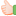A Flash Developer Resource Site

1. ##angle conversion?

Hi folks,

when I'd like to know the angle between two vectors, sometimes I get negative values (radian or degree). How do you convert them so I always get positive values?

Flash seems to have a strange system for calculating angles. I used the following code for doing this, but sometimes I get negative values. Please - can someone help me with this problem?Code:
```public function rangedAngleBetween(otherVector:EuclideanVector):Number
{
var firstAngle:Number;
var secondAngle:Number;

var angle:Number;

firstAngle = Math.atan2(otherVector.position.y, otherVector.position.x);
secondAngle = Math.atan2(position.y, position.x);

angle = secondAngle - firstAngle;

while (angle > Math.PI)
angle -= Math.PI * 2;
while (angle < -Math.PI)
angle += Math.PI * 2;

return angle;
}```Reply With Quote

2. Try setting: var angle:Number; to var angle:uint;Reply With Quote

3. I'll try that. But I'm not sure if that's gonna work...thanks anyway!Reply With Quote

4. Don't know if you are still having trouble with this, but if you are you can always use Math.abs(angle) to change any negative angle into a positive. Also, if you are trying to convert the angle from radians to degrees, you need
Actionscript Code:
`var angleInDegrees:Number = angle*180/Math.PI`
However, if you are going to do something with the angle later, I would suggest not changing it to degrees since you will just have to change it back to radians to use it again. Hope this helps.Reply With Quote

5. ##rotating knob control

Hi David Bernier,

I believe that you can help me with one of the simple Flash AS3 technique, My problem is as follows...........

I want to create a Rotating Control Knob(Circular Dial) to control some paramater using values 0% to 100%.....

I must get the values displayed in a text field when the Knob is rotated ............Reply With Quote

6. Here's a writeup of how Flash handles angles: http://www.calypso88.com/?p=512Reply With Quote

7. Thanks for your answer. But I got the problem solved and now it works like a charm. Problem was that I didn't understand HOW the cos works. So I took an old maths school book where cos was explained. I was confused that it outputs a value between 1 and -1 because I thought it outputs values in radians. That was the mistake I made.Reply With Quote

####Posting Permissions

• You may not post new threads
• You may not post replies
• You may not post attachments
• You may not edit your posts
•

 » Home » Movies » Tutorials » Submissions » Board » Links » Reviews » Feedback » Gallery » Fonts » The Lounge » Sound Loops » Sound FX » About FK » Sitemap